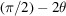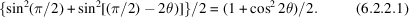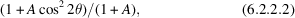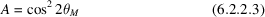International
Tables for
Crystallography
Volume C
Mathematical, physical and chemical tables
Edited by E. Prince

International Tables for Crystallography (2006). Vol. C, ch. 6.2, p. 596

## Section 6.2.2. The polarization factor

H. Lipson,a J. I. Langforda and H.-C. Hub

aSchool of Physics & Astronomy, University of Birmingham, Birmingham B15 2TT, England, and bChina Institute of Atomic Energy, PO Box 275 (18), Beijing 102413, People's Republic of China

### 6.2.2. The polarization factor

| top | pdf |

X-rays are an electromagnetic radiation, and the amplitude with which they are scattered is proportional to the sine of the angle between the direction of the electric vector of the incident radiation and the direction of scattering. Synchrotron radiation is practically plane-polarized, with the electric vector in the plane of the ring, but the radiation from an ordinary X-ray tube is unpolarized, and it may thus be regarded as consisting of two equal parts, half with the electric vector in the plane of scattering, and half with the electric vector perpendicular to this plane. For the latter, the relevant angle is π/2, and for the former it is. The intensity is proportional to the square of the amplitude, so that the polarization factor – really the non-polarization factor – isIf the radiation has been `monochromatized' by reflection from a crystal, it will be partially polarized, and the two parts of the beam will be of unequal intensity. The intensity of reflection then depends on the angular relations between the original, the reflected, and the scattered beams, but in the commonest arrangements all three are coplanar. The polarization factor then becomeswhereandis the Bragg angle of the monochromator crystal. The expression (6.2.2.2)may be substituted for (6.2.2.1)in Table 6.2.1.1whenever appropriate.